Engineering Entrance Sample Papers

GATE Computer Science Paper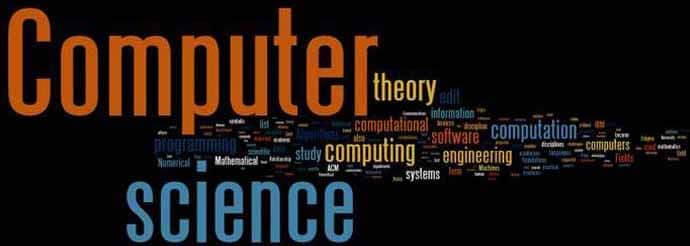GATE CS Exam Pattern: 20 maths questions, 35 questions from Computer Science , 10 aptitude and reasoning questions.

Questions carrying One Mark Each

Question 1:
Consider the following C function
float f,(float x, int y) {
float p, s; int i;
for (s=1,p=1,i=1; i<y; i++) {
p *= x/i;
s+=p;
}
return s;
}
For large values of y, the return value of the function f best approximates
(a)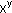(b)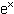(c) ln(1 + x)
(d)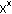Ans: (c)

Question 2:
Consider the polynomial: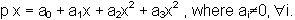The minimum number of multiplications needed to evaluate p on an input x is:
(a) 3
(b) 4
(c) 6
(d) 9
Ans: (a)

Question 3: Which one of the following in NOT necessarily a property of a Group?
(a) Commutativity
(b) Associativity
(c) Existence of inverse for every element
(d) Existence of identity
Ans: (a)

Question 4: For which one of the following reasons does Internet Protocol (IP) use the time-to-live (TTL) field in the IP datagram header?
(a) Ensure packets reach destination within that time
(b) Discard packets that reach later than that time
(c) Prevent packets from looping indefinitely
(d) Limit the time for which a packet gets queued in intermediate routers.
Ans: (c)

Question 5: Consider the following grammar.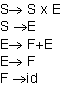Consider the following LR(0) items corresponding to the grammar above.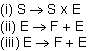Given the items above, which two of them will appear in the same set in the canonical sets-of-items for the grammar?
(a) (i) and (ii)
(b) (ii) and (iii)
(c) (i) and (iii)
(d) None of the above
Ans: (c)

Question 6: A CPU has 24-bit instructions. A program starts at address 300 (in decimal). Which one of the following is a legal program counter (all values in decimal)?
(a) 400
(b) 500
(c) 600
(d) 700
Ans: (c)

Question 7: Let S be an NP-complete problem and Q and R be two other problems not known to be in NP. Q is polynomial time reducible to S and S is polynomial-time reducible to R. Which one of the following statements is true?
(a) R is NP-complete
(b) R is NP-hard
(c) Q is NP-complete
(d) Q is NP-hard
Ans: (c)

Question 8: In the Karnaugh map shown below, X denotes a don’t care term. What is theminimal form of the function represented by the Karnaugh map?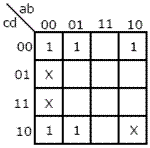(a)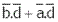(b)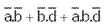(c)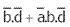(d)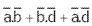Ans: (a)

Question 9: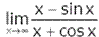equals:

(a) 1
(b) -1

(c)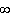(d) –Ans: (a)

Question 10: The most efficient algorithm for finding the number of connected components in an undirected graph on n vertices and m edges has time complexity (a)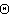(n)
(b)(m)
(c)(m + n)
(d)(mn)
Ans: (c)

Question 11: Which of the following are decidable? I. Whether the intersection of two regular languages is infinite. II. Whether a given context-free language is regular. III. Whether two push-down automata accept the same language. IV. Whether a given grammar is context-free.
(a) I and II
(b) I and IV
(c) II and III
(d) II and IV
Ans: (b)

Question 12: What is the maximum size of data that the application layer can pass on to the TCP layer below?
(a) Any size
(b)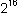bytes-size of TCP header
(c)bytes
(d) 1500 bytes
Ans: (a)

Question 13: Which of the following system calls results in the sending of SYN packets?
(a) socket
(b) bind
(c) listen
(d) connect
Ans: (d)

Question 14: The Breadth First Search algorithm has been implemented using the queue data structure. One possible order of visiting the nodes of the following graph is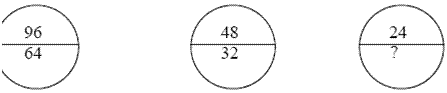(a) MNOPQR
(b) NQMPOR
(c) QMNPRO
(d) QMNPOR
Ans: (c)

Question 15: Newton-Raphson method is used to compute a root of the equation 2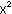-13 = 0 with 3.5 as the initial value. The approximation after one iteration is
(a) 3.575
(b) 3.676
(c) 3.667
(d) 3.607
Ans: (d)

Question 16: A main memory unit with a capacity of 4 megabytes is built using 1M × 1 – bit DRAM chips. Each DRAM chip has 1K rows of cells with 1K cells in each row. The time taken for a single refresh operation is 100 nanoseconds. The time required to perform one refresh operation on all the cells in the memory unit is
(a) 100 nanoseconds
(b) 200 ×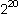nanoseconds
(c) 100 ×nanoseconds
(d) 3200 ×nanoseconds
Ans: (d)

Question 17: P is a 16-bit signed integer. The 2’s complement representation of P is (F87B)16. The 2’s complement representation of 8 × P is
(a) (C3D8)16
(b) (187B)16
(c) (F878)16
(d) (987B)16
Ans: (a)

Question 18: What does the following program print?

#include< stdio.h>
void f( int *p, int * g)
{
p =q;
*p= 2;
}
int i =0, j= 1;
int main ( ){
f(&i, & j);
pr int f (“%d%d n”, i, j) ;
return 0;
}

(a) 2 2
(b) 2 1
(c) 0 1
(d) 0 2
Ans: (b)

Question 19: Which data structure in a compiler is used for managing information about variables and their attributes?
(a) Abstract syntax tree
(b) Symbol table
(c) Semantic stack
(d) Parse table
Ans: (b)

Question 20: Which one of the following is not a client server application?
(a) Internet chat
(b) Web browsing
(c) E-mail
(d) Ping
Ans: (d)

Question 21: Which of the following concurrency control protocols ensure both conflict serializability and freedom from deadlock?
I. 2-phase locking
II. Time-stamp ordering
(a) I only
(b) II only
(c) Both I and II
(d) Neither I nor II
Ans: (b)

Question 22: A system uses FIFO policy for page replacement. It has 4 page frames with no pages loaded to begin with. The system first accesses 100 distinct pages in some order and then accesses the same 100 pages but now in the reverse order. How many page faults will occur?
(a) 196
(b) 192
(c) 197
(d) 195
Ans: (a)

Related: GATE ME Practice Questions

Question 23: K4 and Q3 are graphs with the following structures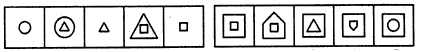Which one of the following statements is TRUE in relation to these graphs?
(a) K4 is planar while Q3 is not
(b) Both K4 and Q3 are planar
(c) Q3 is planar while K4 is not
(d) Neither K4 not Q3 is planar
Ans: (b)

Question 24: A company needs to develop digital signal processing software for one of its newest inventions. The software is expected to have 40000 lines of code. The company needs to determine the effort in person-months needed to develop this software using the basic COCOMO model. The multiplicative factor for this model is given as 2.8 for the software development on embedded systems, while the exponentiation factor is given as 1.20. What is the estimated effort in personmonths?
(a) 234.25
(b) 932.50
(c) 287.80
(d) 122.40
Ans: (a)

Question 25: Which of the following pairs have DIFFERENT expressive power?
(a) Deterministic finite automata (DFA) and Non-deterministic finite automata (NFA)
(b) Deterministic push down automata (DPDA) and Non-deterministic push down automata (NPDA)
(c) Deterministic single-tape Turing machine and Non-deterministic single tape Turing machine
(d) Single-tape Turing machine and multi-tape Turing machine
Ans: (b)

Questions carrying One Mark Each

Question 26: Consider an instruction pipeline with four stages (S1, S2, S3 and S4) each with combinational circuit only. The pipeline registers are required between each stage and at the end of the last stage. Delays for the stages and for the pipeline registers are as given in the figure.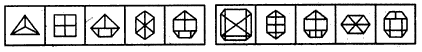What is the approximate speed up of the pipeline in steady state under ideal conditions when compared to the corresponding non-pipeline implementation?
(a) 4.0
(b) 2.5
(c) 1.1
(d) 3.0
Ans: (b)

Question 27: An 8KB direct mapped write-back cache is organized as multiple blocks, each of size 32-bytes. The processor generates 32-bit addresses. The cache controller maintains the tag information for each cache block comprising of the following. 1 Valid bit 1 Modified bit As many bits as the minimum needed to identify the memory block mapped in the cache. What is the total size of memory needed at the cache controller to store metadata (tags) for the cache? (a) 4864 bits
(b) 6144 bits
(c) 6656 bits
(d) 5376 bits
Ans: (d)

Question 28: Database table by name Loan_Records is given below.

 Borrower Bank_Manager Loan_ Amount Ramesh Sunderajan 10000.00 Suresh Ramgopal 5000.00 Mahesh Sunderajan 7000.00

What is the output of the following SQL query? SELECT count(*) FROM( (SELECT Borrower. Bank_Manager FROM Loan_Records) AS S NATURAL JOIN (SELECT Bank_Manager, Loan_Amount FROM Loan_Records) AS T );
(a) 3
(b) 9
(c) 5
(d) 6
Ans: (c)

Question 29: Consider the following table of arrival time and burst time for three processes P0, P1 and P2.

 Process Arrival time Burst Time P0 0 ms 9 ms P1 1 ms 4 ms P2 2 ms 9 ms

The pre-emptive shortest job first scheduling algorithm is used. Scheduling is carried out only at arrival or completion of processes. What is the average waiting time for the three processes?
(a) 5.0 ms
(b) 4.33 ms
(c) 6.33 ms
(d) 7.33 ms
Ans: (a)

Question 30: Let w be any string of length n in {0, 1}*. Let L be the set of all substrings of w. What is the minimum number of states in a non-deterministic finite automaton that accepts L?
(a) n – 1
(b) n
(c) n + 1
(d) 2n – 1
Ans: (c)

Question 31: The following program consists of 3 concurrent processes and 3 binary semaphores. The semaphores are initialized as S0 = 1, S1 = 0, S2 = 0.

 Process P0 Process P1 Process P2 while (true) { wait (S0); print ‘0’ release (S1); release (S2); Wait (S1); Release (S0); Wait (S2); Release (S0);

How many times will process P0 print ‘0’?
(a) At least twice
(b) Exactly twice
(c) Exactly thrice
(d) Exactly once
Ans: (a)

Question 32: Suppose computers A and B have IP addresses 10.105.1.113 and 10.105.1.91 respectively and they both use the same net mask N. Which of the values of N given below should not be used if A and B should belong to the same network?
(a) 255.255.255.0
(b) 255.255.255.128
(c) 255.255.255.192
(d) 255.255.255.224
Ans: (d)

Question 33: What is the return value of the function foo when it is called as foo (345, 10) ?
(a) 345
(b) 12
(c) 5
(d) 3
Ans: (b)

Question 34: If at some instance prior to the occurrence of the clock edge, P. Q and R have a value 0, 1 and 0 respectively, what shall be the value of PQR after the clock edge?
(a) 000
(b) 001
(c) 010
(d) 011
Ans: (d)

Question 35: Four matrices M1, M2, M3 and M4 are dimensions p × q, q × r, r × s and s × t respectively can be multiplied in several ways with different number of total scalar multiplications. For example When multiplied as ((M1 × M2) × (M3 × M4)) the total number of scalar multiplications is pqr + rst + prt. When multiplied as (((M1 × M2) × M3) × M4), the total number of scalar multiplications is pqr + prs + pst.

If p = 10, q = 100, r = 20, s = 5 and t = 80, then the minimum number of scalar multiplications needed is
(a) 248000
(b) 44000
(c) 19000
(d) 25000
Ans: (c)

Question 36: Consider the matrix as given below.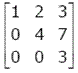Which one of the following provides the CORRECT values of eigenvalues of the matrix?
(a) 1, 4, 3
(b) 3, 7, 3
(c) 7, 3, 2
(d) 1, 2, 3
Ans: (a)

Question 37: Which of the following graphs has an Eulerian circuit?
(a) Any k-regular graph where k is an even number.
(b) A complete graph on 90 vertices.
(c) The complement of a cycle on 25 vertices.
(d) None of the above
Ans: (a)

Related: GATE EC Practice Question Paper

Question 38: Suppose only one multiplexer and one inverter are allowed to be used to implement any Boolean function of n variables. What is the minimum size of the multiplexer needed?
(a) 2n line to 1 line
(b)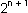line to 1 line
(c)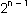line to 1 line
(d) n line to 1 line
Ans: (c)

Question 39:

The control signal functions of a 4-bit binary counter are given below (where X is “don’t care”):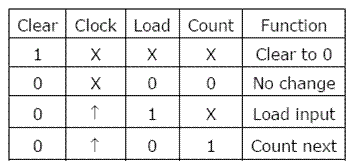The counter is connected as follows: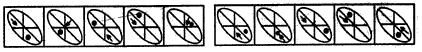Assume that the counter and gate delays are negligible. If the counter starts at 0, then it cycles through the following sequence:
(a) 0, 3, 4
(b) 0, 3, 4, 5
(c) 0, 1, 2, 3, 4
(d) 0, 1, 2, 3, 4, 5
Ans: (d)

Question 40: Consider a pipelined processor with the following four stages: IF: Instruction Fetch ID: Instruction Decode and Operand Fetch EX: Execute WB: Write Back The IF, ID and WB stages take one clock cycle each to complete the operation. The number of clock cycles for the EX stage depends on the instruction. The ADD and SUB instructions need 1 clock cycle and the MUL instruction needs 3 clock cycles in the EX stage. Operand forwarding is used in the pipelined processor. What is the number of clock cycles taken to complete the following sequence of instructions? ADD           R2, R1, R0         R2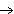R1 + R0 MUL           R4, R3, R2         R4R3 × R2 SUB           R6, R5, R4          R6R5 – R4
(a) 7
(b) 8
(c) 10
(d) 14
Ans: (b)

Question 41: A complete n-ary tree is a tree in which each node has n children or no children. Let I be the number of internal nodes and L be the number of leaves in a complete n-ary tree. If L = 41, and I = 10, what is the value of n? (a) 3
(b) 4
(c) 5
(d) 6
Ans: (c)

Question 42: Which of the following is TRUE about formulae in Conjunctive Normal Form?
(a) For any formula, there is a truth assignment for which at least half the clauses evaluate to true.
(b) For any formula, there is a truth assignment for which all the clauses evaluate to true.
(c) There is a formula such that for each truth assignment, at most one-fourth of the clauses evaluate to true.
(d) None of the above.
Ans: (b)

Question 43:

Consider the following two statements:
P: Every regular grammar is LL (1)
Q: Every regular set has a LR(1) grammar
Which of the following is TRUE?

(a) Both P and Q are true
(b) P is true and Q is false
(c) P is false and Q is true
(d) Both P and Q are false
Ans: (b)

Question 44: Two processes, P1 and P2, need to access a critical section of code. Consider the following synchronization construct used by the processes: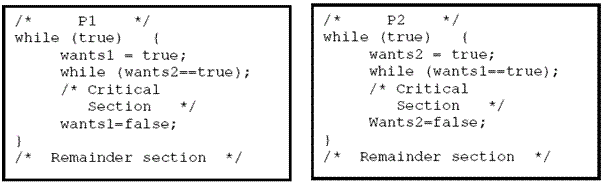Here, wants1 and wants2 are shared variables, which are initialized to false. Which one of the following statements is TRUE about the above construct?
(a) It does not ensure mutual exclusion.
(b) It does not ensure bounded waiting.
(c) It requires that processes enter the critical section in strict alternation.
(d) It does not prevent deadlocks, but ensures mutual exclusion.
Ans: (d)

Question 45: Information about a collection of students is given by the relation studinfo (studId, name, sex). The relation enroll (studId, courseId) gives which student has enrolled for (or taken) what course(s). Assume that every course is taken by at least one male and at least one female student. What does the following relational algebra expression represent?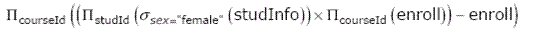(a) Courses in which all the female students are enrolled.
(b) Courses in which a proper subset of female students are enrolled.
(c) Courses in which only male students are enrolled.
(d) None of the above.
Ans: (b)

Question 46: Which one of the following statements if FALSE?
(a) Any relation with two attributes is in BCNF
(b) A relation in which every key has only one attribute is in 2NF
(c) A prime attribute can be transitively dependent on a key in a 3 NF relation.
(d) A prime attribute can be transitively dependent on a key in a BCNF relation.
Ans: (d)

Question 47: In a token ring network the transmission speed is 10bps and the propagation speed is 200 metres / Rs. The 1-bit delay in this network is equivalent to:
(a) 500 metres of cable.
(b) 200 metres of cable.
(c) 20 metres of cable.
(d) 50 metres of cable.
Ans: (c)

Question 48: Match the following:

(P) SMTP – (1) Application layer
(Q) BGP – (2) Transport layer
(R) TCP – (3) Data link layer
(S) PPP – (4) Network layer
(5) Physical layer
(a) P – 2 Q – 1 R – 3 S – 5
(b) P – 1 Q – 4 R – 2 S – 3
(c) P – 1 Q – 4 R – 2 S – 5
(d) P – 2 Q – 4 R – 1 S – 3
Ans: (b)

Question 49: Consider evaluating the following expression tree on a machine with load-store architecture in which memory can be accessed only through load and store instructions. The variables a, b, c, d and e are initially stored in memory. The binary operators used in this expression tree can be evaluated by the machine only when the operands are in registers. The instructions produce result only in a register. If no intermediate results can be stored in memory, what is the minimum number of registers needed to evaluate this expression?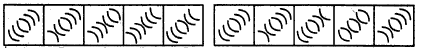(a) 2
(b) 9
(c) 5
(d) 3
Ans: (d)

Question 50: A deck of 5 cards (each carrying a distinct number from 1 to 5) is shuffled thoroughly. Two cards are then removed one at a time from the deck. What is the probability that the two cards are selected with the number on the first card being one higher than the number on the second card?
(a) 1/5
(b) 4/25
(c) 1/4
(d) 2/5
Ans: (a)

Question 51: On a non-pipelined sequential processor, a program segment, which is a part of the interrupt service routine, is given to transfer 500 bytes from an I/O device to memory.

Initialize the count to 500
LOOP: Load a byte from device
Decrement the count
If count != 0 go to LOOP

Assume that each statement in this program is equivalent to a machine instruction which takes one clock cycle to execute if it is a non-load/store instruction. The load-store instructions take two clock cycles to execute.

The designer of the system also has an alternate approach of using the DMA controller to implement the same transfer. The DMA controller requires 20 clock cycles for initialization and other overheads. Each DMA transfer cycle takes two clock cycles to transfer one byte of data from the device to the memory.

What is the approximate speedup when the DMA controller based design is used in place of the interrupt driven program based input-output?

(a) 3.4
(b) 4.4
(c) 5.1
(d) 6.7
Ans: (a)

For next 2 Question:

Consider a network with five nodes, N1 to N5, as shown below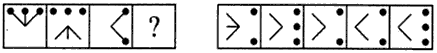The net work uses a Distance Vector Routing protocol. Once the routes have stabilized, the distance vectors at different nodes are as following

N1: (0, 1, 7, 8, 4)
N2 : (1, 0, 6, 7, 3)
N3 : (7, 6, 0, 2, 6)
N4 : (8, 7, 2, 0, 4)
N5 : (4, 3, 6, 4, 0)

Each distance vector is the distance of the best known path at that instance to nodes, N1 to N5, where the distance to itself is 0. Also, all links are symmetric and the cost is identical in both directions. In each round, all nodes exchange their distance vectors with their respective neighbors. Then all nodes update their distance vectors. In between two rounds, any change in cost of a link will cause the two incident nodes to change only that entry in their distance vectors

Question 52:

The cost of link N2-N3 reduces to 2 in (both directions). After the next round of updates, what will be the new distance vector at node, N3?
(a) (3, 2, 0, 2, 5)
(b) (3, 2, 0, 2, 6)
(c) (7, 2, 0, 2, 5)
(d) (7, 2, 0, 2, 6)
Ans: (a)

Question 53: After the update in the previous question, the link N1 – N2 goes down. N2 will reflect this change immediately in its distance vector as cost,. After the NEXT ROUND of update, what will be the cost to N1 in the distance vector of N3?
(a) 3
(b) 9
(c) 10
(d)Ans: (c)

For Question 52 and 53 :

Consider a network with 6 routers R1 to R6 connected with links having weights as shown in the following diagram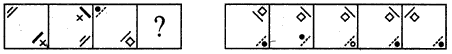Question 54: All the routers use the distance vector based routing algorithm to update their routing tables. Each router starts with its routing table initialized to contain an entry for each neighbour with the weight of the respective connecting link. After all the routing tables stabilize, how many links in the network will never be used for carrying any data?
(a) 4
(b) 3
(c) 2
(d) 1
Ans: (b)

Question 55: Suppose the weights of all unused links in the previous question are changed to 2 and the distance vector algorithm is used again until all routing tables stabilize. How many links will now remain unused?
(a) 0
(b) 1
(c) 2
(d) 3
Ans: (c)

Related: Computer Science Career Guide

Questions carrying One Mark Each

Question 56: Choose the most appropriate word from the options given below to the complete the following sentence: His rather casual remarks on politics ___________ his lack of seriousness about the subject.
(b) belied
(c) betrayed
(d) suppressed
Ans: (a)

Question 57: If Log (P) = (1/2)Log (Q) = (1/3) Log (R), then which of the following options is TRUE?
(a) P= QR
(b)  Q= PR
(c) Q= RP
(d)  R = PQ2
Ans: (b)

Question 58: 25 persons are in a room. 15 of them play hockey, 17 of them play football and 10 of them play both hockey and football. Then the number of persons playing neither hockey nor football is:
(a) 2
(b) 17
(c) 13
(d) 3
Ans: (d)

Question 59: Choose the word from the options given below that is most nearly opposite in meaning to the given word: Amalgamate
(a) Merge
(b) Split
(c) Collect
(d) Separate
Ans: (b)

Question 60: Choose the most appropriate word from the options given below to complete the following sentence. If you are trying to make a strong impression on your audience, you cannot do so by being understated, tentative or ___.
(a) Hyperbolic
(b) Restrained
(c) Argumentative
(d) Indifferent
Ans: (b)

Questions carrying Two Marks Each

Question 61: P, Q, R and S are four types of dangerous microbes recently found in a human habitat. The area of each circle with its diameter printed in brackets represents the growth of a single microbe surviving human immunity system within 24 hours of entering the body. The danger to human beings varies proportionately with the toxicity, potency and growth attributed to a microbe shown in the figure below.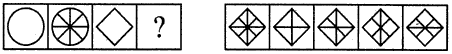A pharmaceutical company is contemplating the development of a vaccine against the most dangerous microbe. Which microbe should the company target in its first attempt?
(a) P
(b) Q
(c) R
(d) S
Ans: (d)

Question 62: The variable cost (V) of manufacturing a product varies according to the equation V= 4q, where q is the quantity produced. The fixed cost (F) of production of same product reduces with q according to the equation F = 100/q. How many units should be produced to minimize the total cost (V+F)?
(a) 5
(b) 4
(c) 7
(d) 6
Ans: (a)

Question 63: Modern warfare has changed from large scale clashes of armies to suppression of civilian populations. Chemical agents that do their work silently appear to be suited to such warfare; and regretfully, there exist people in military establishments who think that chemical agents are useful tools for their cause.
Which of the following statements best sums up the meaning of the above passage:
(a) Modern warfare has resulted in civil strife.
(b) Chemical agents are useful in modern warfare.
(c) Use of chemical agents in warfare would be undesirable.
(d) People in military establishments like to use chemical agents in war.
Ans: (c)

Question 64: Given digits 2, 2, 3, 3, 4, 4, 4, 4 how many distinct 4 digit numbers greater than 3000 can be formed?
(a) 50
(b) 51
(c) 52
(d) 54
Ans: (b)

Question 65: A container originally contains 10 litres of pure spirit. From this container 1 litre of spirit is replaced with 1 litre of water. Subsequently, 1 litre of the mixture is again replaced with 1 litre of water and this process is repeated one more time. How much spirit is now left in the container?
(a) 7.58 litres
(b) 7.84 litres
(c) 7 litres
(d) 7.29 litres
Ans: (d)